# Division + ratio - math problems

#### Number of problems found: 104

• Write 4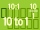Write each ratio as a fraction of whole numbers: a 4.8 to 11.2 6) 2.7 to 0.54
• A sumA sum of money is shared between Peter, John and Henry in the ratio 2:3:5. a) express Henry's share as fraction of John's share. b) what fraction of the whole sum of money is John's share?
• Aspect ratioDetermine the ratio of the length to the width of a picture frame that is 12 3/4 inches long and 8 1/2 inches wide.
• Math club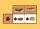A math club has 25 members of which 11 are male and the rest are female. What is the ratio of all male to all club?
• An orchard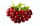In an orchard, there are 28 apple trees, 27 orange trees, and 14 cherry trees. What is the ratio of apple trees to cherry trees?
• Construct 8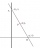Construct an analytical geometry problem where it is asked to find the vertices of a triangle ABC: the vertices of this triangle must be the points A (1,7) B (-5,1) C (5, -11). the said problem should be used the concepts of: distance from a point to a li
• An amusement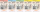An amusement park sold 40 child tickets. The other 10 tickets it sold were adult tickets. What is the ratio of the number of adult tickets to the number of child tickets?
• Carla 2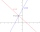Carla is renting a canoe, it cost \$80 for 2 hours and \$110 for 4 hours. What is the rate of change for this situation?
• ErikThree friends Peter, Erik and Milan shared 420 euros in a ratio of 1: 2: 3. How many euros did Erik get?
• TogetherThe three friends divided the balls in a ratio of 6: 5: 4. Some two of them got a total of 126 balls. How many balls were there together?
• Line segment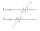Line segment AB is 8 cm long. Divide it in a ratio of 2: 3.
• 120 nuts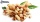Divide 120 nuts in a ratio of 4: 6.
• Prime divisorsFind 2/3 of the sum's ratio and the product of all prime divisors of the number 120.
• Coke and coal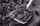20.1 tons of coke is produced from 30 tons of black coal. How much coke is made from 1 kilogram of coal?
• Two villages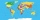Two villages are 11 km and 500 m away. On the map, their distance is determined by a 5 cm long line. Find the scale of the map.
• Volume of sphereHow many times does the volume of a sphere increase if its radius increases 2 ×?
• The string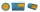They cut 113 cm from the string and divided the rest in a ratio of 5: 6.5: 8: 9.5. The longest part measured 38 cm. Find the original length of the string.
• Divide in ratioLine segment AB 12 cm long divide in a ratio of 5: 3. How long are the individual parts?
• Father and daughterFather is 36 years old, daughter is 20 years less. What will be the ratio between them when they are 10 years more?
• Two bodiesThe rectangle with dimensions 8 cm and 4 cm is rotated 360º first around the longer side to form the first body. Then, we similarly rotate the rectangle around the shorter side b to form a second body. Determine the ratio of surfaces of the first and seco

Do you have an exciting math question or word problem that you can't solve? Ask a question or post a math problem, and we can try to solve it.

We will send a solution to your e-mail address. Solved examples are also published here. Please enter the e-mail correctly and check whether you don't have a full mailbox.

Check out our ratio calculator. Division Problems. Ratio - math problems.